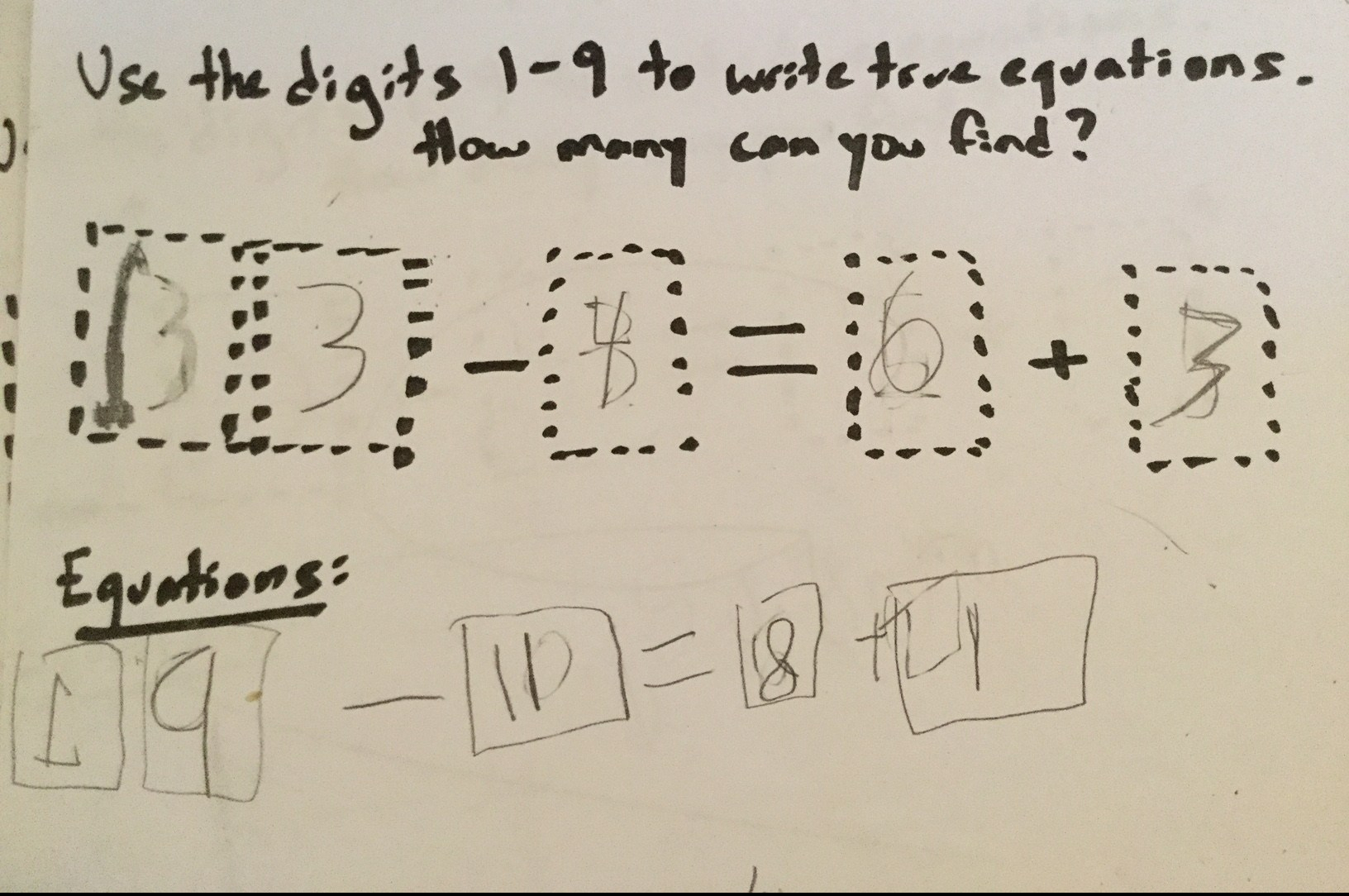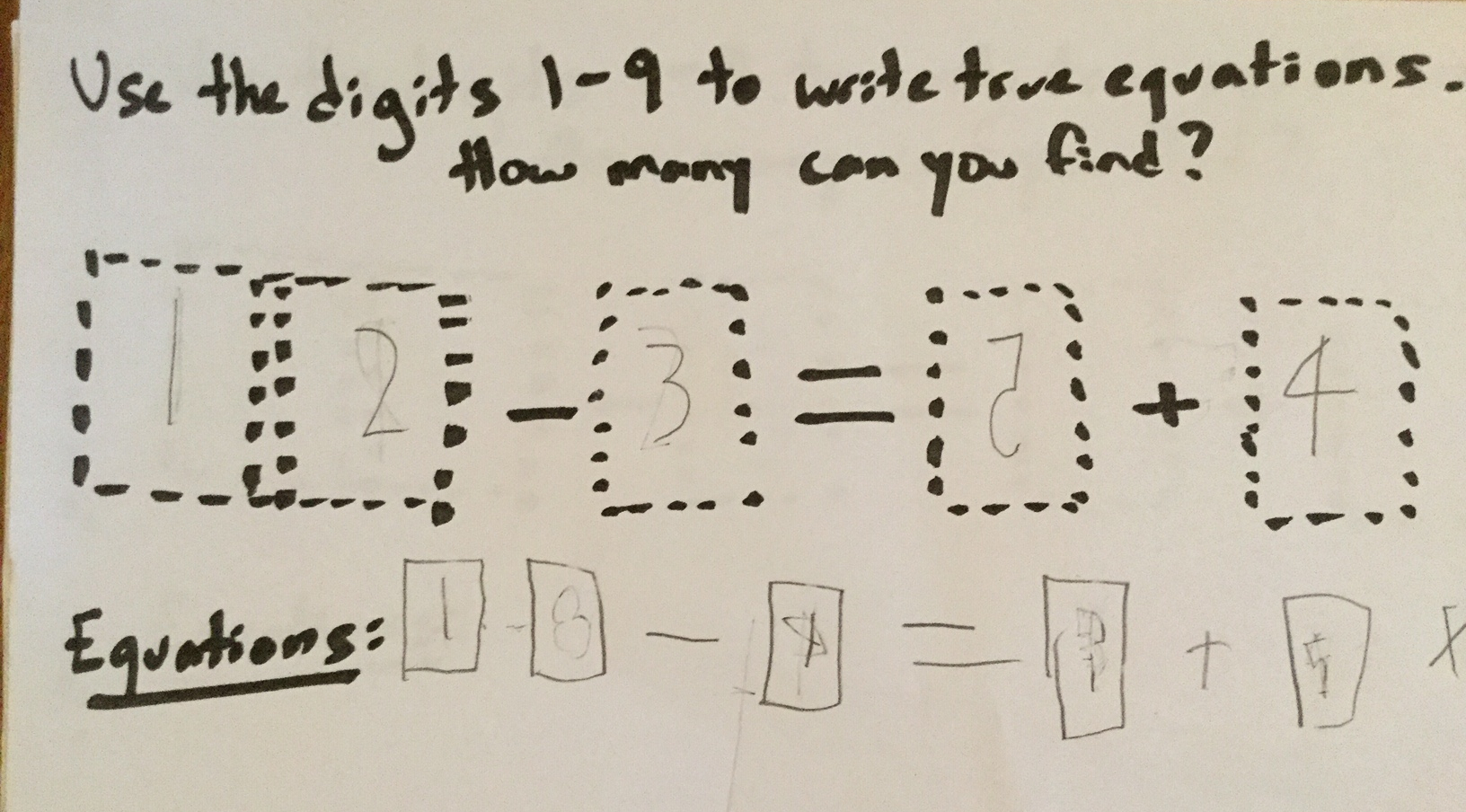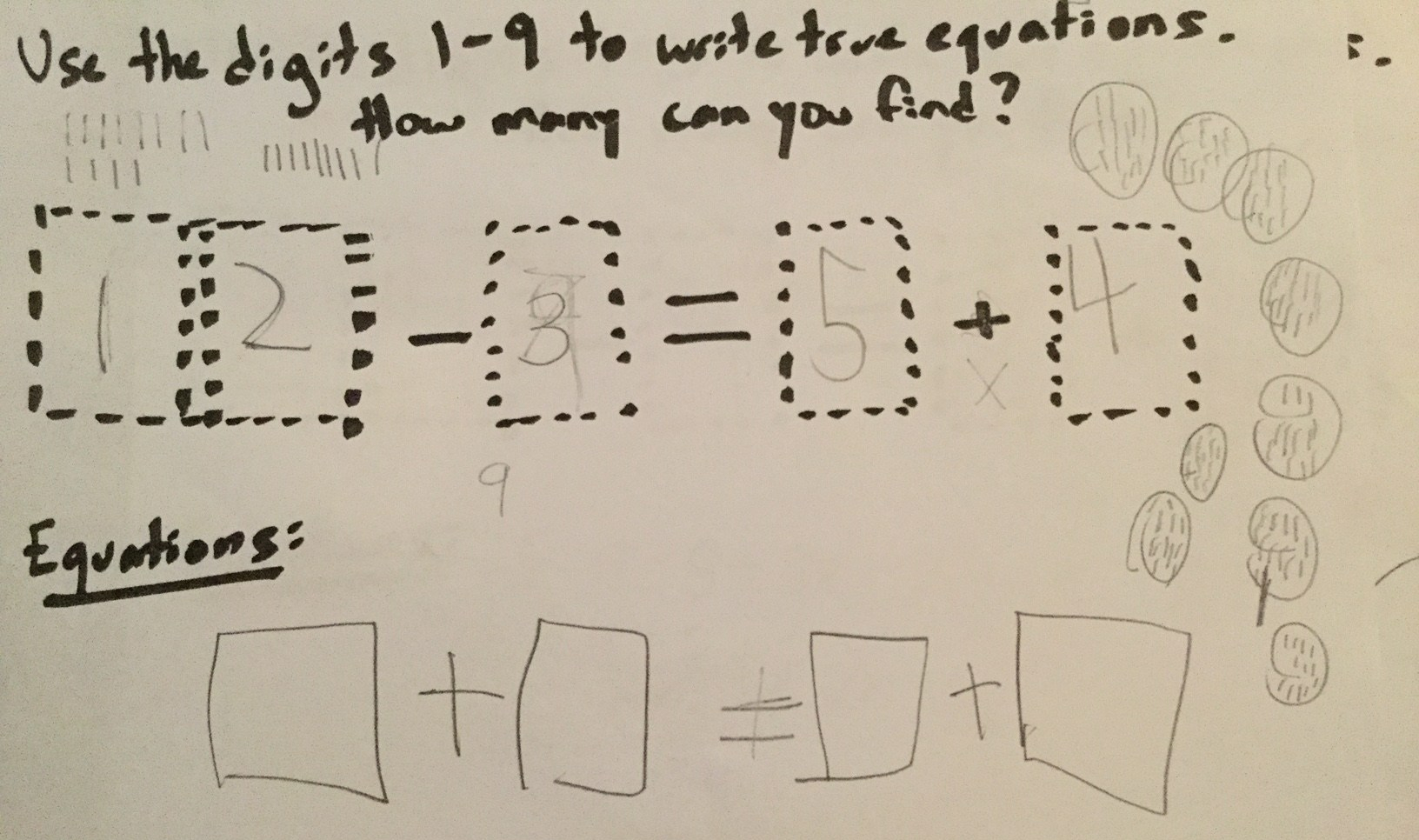# Equations and Equivalence in 3rd Grade

So I was stupidly mouthing off online to some incredibly serious researchers about equivalence and the equals sign and how it’s not that hard of a topic to teach when — OOPS! — my actual teaching got in the way.

I had done the right thing. In my 3rd Grade class I wanted to introduce “?” as a symbol for an unknown so I put up some equations on the board:

15 = ? x 5

3 + ? = 10

10 + 3 = 11 + ?

And I was neither shocked, nor did I blink, when a kid told me that the last equation didn’t make any sense. Ah, I thought, time to nip this in the bud.

I listened to the child and said I understood, but that I would like to share how it does make sense. I asked whether anyone knew what the equals sign meant, and one kid says “makes” and the next said “the same as.” Wonderful, I said, because that last equation is just saying the left side equals the same as the right side. So what number would make them the same? 2? Fantastic, let’s move on.

Then, the next day, I put a problem on the board:

5+ 10 = ___ + 5

And you know what comes next, right? Consensus around the room is that the blank is 15. “But didn’t we say yesterday that the equals sign means ‘the same as’?” I asked. A kid raised her hand and explained that it did mean that, but the answer should still be 15. Here’s how she wanted us to read the equation, as a run on:

(5 + 10 =  15 ) + 5

Two things were now clear to me. First, that my pride in having clearly and decisively taken care of this issue was misguided. I needed to do more and dig into this more deeply.

The second thing is that isn’t this interesting? You can have an entirely correct understanding of the equals sign and still make the same “classic” mistakes interpreting an actual equation.

I think this helps clear up some things that I was muddling in my head. When people talk about the need for kids to have a strong understanding of equivalence they really are talking about quite a few different things. Here are the two that came up above:

• The particular meaning of the equals sign (and this is supposed to entail that an equation can be written left-to-right or right-to-left, i.e. it’s symmetric)
• The conventional ways of writing equations (e.g. no run ons, can include multiple operations and terms on each side)

But then this is just the beginning, because frequently people talk about a bunch of other things when talking about ‘equivalence.’ Here are just a few:

• You can do the same operations to each side (famously useful for solving equations)
• You can manipulate like terms on one side of an equation to create a true equation (10 + 5 can be turned into 9 + 6 can be turned into 8 + 7; 8 x 7 can be turned into 4 x 14; 3(x + 4) can be turned into 3x + 12, etc.)

When a kid can’t solve 5 + 10 = ___ + 9 correctly or easily using “relational understanding,” this is frequently blamed on a kid’s understanding of the equals sign, equivalence or the particular ways of relating 5 + 10 to __ + 9. But now I’m seeing clearly that these are separate things, and some tend to be easier for kids than others.

So, this brings us to the follow-up lesson with my 3rd Graders.

I started as I usually do in this situation, by avoiding the equals sign. I find that a double arrow serves this purpose well, so I put up an arrow relationship on the board:

2 x 6 <–> 8 + 4

I pointed out that 2 x 6 makes 12 and so does 8 + 4. Could the kids come up with other things like this, I asked?

They did. I didn’t grab a picture, but I was grateful that all sorts of things came up. Kids were mixing operations nicely, like 12 – 2 <–> 5 + 5, in general it felt like this was not hard, kids knew exactly what I meant and could generate lots of ideas.

My next move was to pause and introduce the equals sign into this conversation. Would anyone mind if I replaced that double arrow with an equals sign? This is just what the equal sign means, anyway. No problem, that went fine also.

Kids were even introducing great examples like 1 x 2 = 2 x 1, or 12 = 12. Wonderful.

Then, I introduced the task of the day, in the style of Open Middle (R) (TM) (C):Yeah, I quickly handwrote it with a sharpie. It was that sort of day.

I carefully explained the constraints. 10 – 2 + 7 + 1 was a true equation, but wouldn’t work for this puzzle. Neither would 15 – 5 = 6 + 4. And then I gave the kids time to search for solutions, as many as they could find.

Bla bla, most kids were successful, others had trouble getting started but everyone eventually had some success. Here are some pictures of students who make me look good:Here is a picture of a student who struggled, but eventually found a solution:Here is a picture of the student from the class I was most concerned with. You can see the marks along his page as he tries to handle things like 12 – 9 as he tries subtracting different numbers from 12. I think there might have been some multiplying happening on the right side, not sure why. Anyway:The thing is that just the day before, this last student had almost broken down in frustration over his inability to make sense of these “unconventional” equations. So this makes me look kind of great — I did it! I taught him equivalence, in roughly a day. Tada.

But I don’t think that this is what’s going on. The notion of two different things being equal, that was not hard for him. In fact I don’t think that notion is difficult for very many students at all — kids know that different additions equal 10. And it was not especially difficult for this kid to merge that notion of equivalence with the equals sign. Like, no, he did not think that this was what the equals sign meant, but whatever, that was just on the basis of what he had thought before. It’s just a convention. I told him the equals sign meant something else, OK, sure. Not so bad either.

The part that was very difficult for this student, however, was subtracting stuff from 12.

Now this is what I think people are talking about when they talk about “relational understanding.” It’s true — I really wish this student knew that 10 + 2 <–> 9 + 3, and so when he saw 12 he could associate that with 10 + 2 and therefore quickly move to 9 + 3 and realize that 12 – 3 = 9. I mean, that’s what a lot of my 3rd Graders do, in not so many words. That is very useful.

So to wrap things up here are some questions and some provisional answers:

Q: Is it hard to teach or learn the concept of equivalence.

A: No.

Q: Is it hard to teach the equals sign and its meaning?

A: It’s harder, but this is all conventional. If you introduce a new symbol like “<–>” I don’t think kids trip up as much. They sometimes have to unlearn what they’ve inferred from prior experiences that were too limited (i.e. always putting the result on the right side). So you’re not doing kids any favors by doing that, it’s good to put the equations in a lot of different forms, pretty much as soon as kids see equations from the first time in K or 1st Grade. I mean why not?

Q: If kids don’t learn how equations conventionally work will that trip them up later in algebra?

A: Yes. But all of my kids find adding and subtracting itself to be more difficult than understanding these conventions. My sense is that you don’t need years to get used to how equations work. You need, like, an hour or two to introduce it.

Q: Does this stuff need to be taught early? Is algebra too late to learn how equations work?

A: I think kids should learn it early, but it’s not too late AT ALL if they don’t.

I have taught algebra classes in 8th and 9th Grade where students have been confused about how equations work. My memories are that this was annoying because I realized too late what was going on and had to backtrack. But based on teaching this to younger kids, I can’t imagine that it’s too late to teach it to older students.

I guess it could be possible that over the years it gets harder to shake students out of their more limited understanding of equations because they reinforce their theory about equations and the equals symbol. I don’t know.

I see no reason not to teach this early, but I think it’s important to keep in mind that in middle school we tell kids that sometimes subtracting a number makes it bigger and that negative exponents exist. Kids can learn new things in later years too.

Q: So what makes it so hard for young kids to handle equations like 5 + 10 = 6 + __?

A: It’s definitely true that kids who don’t understand how to read this sort of equation will be unable to engage at all. But the relational thinking itself is the hardest part to teach and learn, it seems to me.

Here is a thought experiment. What if you had a school or curriculum that only used equal signs and equations in the boring, limited way of “5 + 10 = ?” and “6 x ? = 12” throughout school, but at the same time taught relational thinking using <–> and other terminology in a deep and effective way? And then in 8th Grade they have a few lessons teaching the “new” way of making sense of the equals sign? Would that be a big deal? I don’t know, I don’t think so.

Q: There is evidence that suggests learning various of the above things helps kids succeed more in later algebra. Your thoughts?

A: I don’t know! It seems to me that if something makes a difference for later algebra, it has to be either the concept of equivalence, the conventions of equations, or relational thinking.

I think the concept of equivalence is something every kid knows. The conventions of equations aren’t that hard to learn, I think, but they really only do make sense if you connect equations to the concept of equivalence. The concept of equivalence explains why equations have certain conventions. So I get why those two go together. But could that be enough to help students with later algebra experiences? Maybe. Is it because algebra teachers aren’t teaching the conventions of equations in their classes? Would there still be an advantage from early equation experience if algebra teachers taught it?

In the end, it doesn’t matter much because young kids can learn it and so why bother not teaching it to them? Can’t hurt, only costs you an hour or two.

But the big other thing is relational thinking. Now there is no reason I think why relational thinking has to take place in the context of equations. You COULD use other symbols like double arrows or whatever. But math already has this symbol for equivalence, so you might as well teach relational thinking about addition/subtraction/multiplication/division in the context of equations. And that’s some really tricky, really important mathematics to learn. A kid being able to understand that 2 x 14 is equal to 4 x 7 is important stuff.

It’s important for so many reasons, for practically every reason that arithmetic is the foundation of algebra. I can’t list them now — but it goes beyond equations, is my point. Relational thinking (e.g. how various additions relate to each other) is huge and hugely important.

Would understanding the conventions of the equals sign and equations make a difference in the absence of experiences that help kids gain relational understanding? Do some kids start making connections on their own when they learn ways of writing equations? Does relational understanding instruction simply fail because kids don’t understand what the equations their teachers are using mean?

I don’t know.

## 2 thoughts on “Equations and Equivalence in 3rd Grade”

1.John Golden says:

You don’t know, but you followed your intuition and clues to a lesson that directly impacted building an understanding. Really great teaching, in my opinion. The ironic twitter conversation is just the special sauce.

2.Ben Blum-Smith says:

Thank you (as usual) for the careful and thoughtful working-through of this juicy stuff.

I think there is a fourth capacity students need to use in algebra, that I read as different from the concept of equivalence, the conventions governing the = sign, and relational thinking, as you’re glossing them, that might be going on here, i.e. that I conjecture is implicated in the research finding that kids who understand the = sign as signifying equivalence have an easier go of algebra later. I suppose equivalence and/or relational thinking could be glossed so as to encompass it, but I don’t really think that does it justice.

It is related to the “equation as process” vs. “equation as object” distinction you draw here, and relatedly to the idea of “encapsulation” that Ed Dubinsky writes about. It’s the facility to move back and forth between the views of an equation as a piece of information telling you something about its constituent parts, on the one hand, vs. an equation as an object that can be subjected to formal manipulations that preserve this information.

(I started to think about this when Cognitively_Accessible_Math probed something I said on twitter about my experience with kids who think in terms of “undoing” when solving equations not really buying my attempts to sell to them that what they were actually doing was doing the same thing to both sides.)

Anyway this facility to move back and forth between these two points on view on an equation, and, maybe as a prerequisite, the facility of taking the second point of view (equation-as-object-that-can-be-manipulated-in-an-info-preserving-way) at all, strike me as deep, not-easy-to-learn things that are directly implicated in algebra success and may well be correlated with aspects of the student’s early thinking about the = sign. FWIW. I have no conjecture to assay at this point about the structure/mechanism of that correlation, but maybe it’s worth keeping this possibility in mind while sorting all this out?